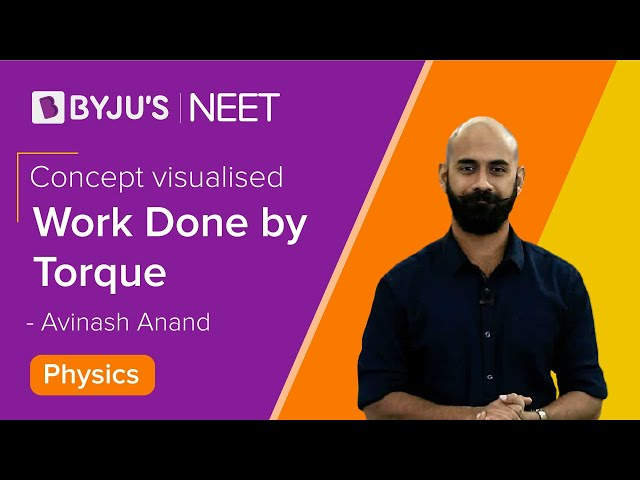Jet Set Go! All about Aeroplanes Jet Set Go! All about Aeroplanes

# Relation between Torque and Power

Torque is the rotational equivalence of linear force. Power is the rate of doing work. The relation between torque and power is directly proportional to each other. The power of a rotating object can be mathematically written as the scalar product of torque and angular velocity.

### Power Formula

 $$\begin{array}{l}P=\tau .\omega\end{array}$$

Where,

• P is the power (work done per unit time)
• τ is the torque (rotational ability of a body)
• ω is the angular velocity(rate of change of angular displacement)
• . represent the dot product or scalar product

The above equation can be rearranged to compute the torque required to achieve given angular velocity and power. The torque injects power, and it purely depends on instantaneous velocity.

## Relationship Between Torque and Power

To derive the relation between torque and Power, compare the linear equivalent for any rotatory motion. The linear displacement is the distance covered at the circumference of the rotation and is given by the product of the angle covered and radius. The linear velocity is given by the product of radius and angular velocity. And linear distance is given by the product of linear velocity and time.

∴ Linear distance = radius × angular velocity × time

Torque makes an object undergo rotational motion. It is expressed as-

$$\begin{array}{l}Force=\frac{Torque}{Radius}\end{array}$$

Thus,

$$\begin{array}{l}Power=\frac{Force\times Linear\;distance}{Time}\end{array}$$
$$\begin{array}{l}Power=\frac{\left ( \frac{Torque}{Radius} \right )\times Radius \times Angular \;velocity \times Time}{Time}\end{array}$$
$$\begin{array}{l}Power=Torque\times Angular\;velocity\end{array}$$

Hope you understood the relation and conversion between the Power and the Torque of a rotating object.

Physics Related Topics:

## To learn about work done by torque, click on the video belowStay tuned with BYJU’S for more such interesting articles. Also, register to “BYJU’S – The Learning App” for loads of interactive, engaging Physics-related videos and unlimited academic assistance.

Test Your Knowledge On Relation Between Torque And Power!